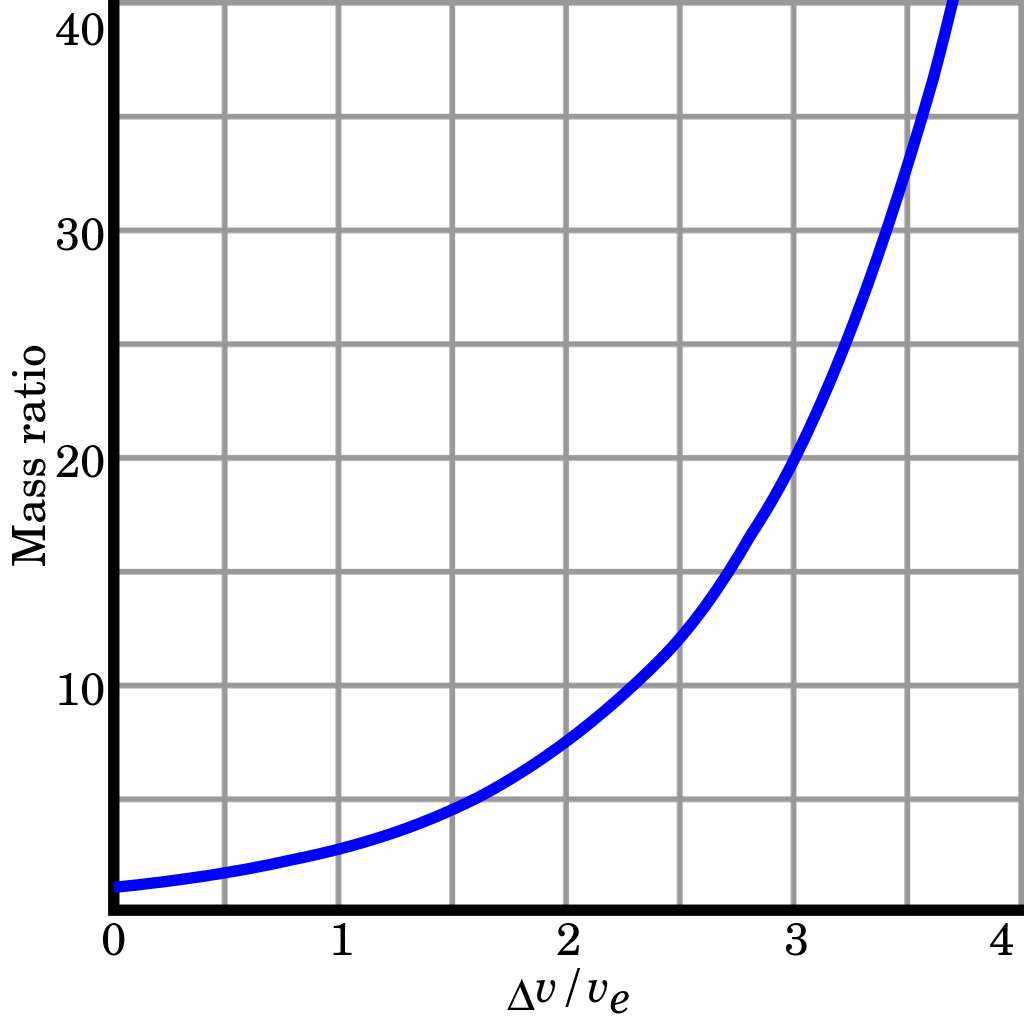## Tsiolkovsky rocket equation

The Tsiolkovsky rocket equation, or ideal rocket equation, describes the motion of vehicles that follow the basic principle of a rocket: a device that can apply acceleration to itself (a thrust) by expelling part of its mass with high speed and move due to the conservation of momentum. The equation relates the delta-v (the maximum change of speed of the rocket if no other external forces act) with the effective exhaust velocity and the initial and final mass of a rocket (or other reaction engine).